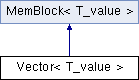HLIBpro  2.9.1
Vector< T_value > Class Template Reference

Standard vector in basic linear algebra, i.e. BLAS/LAPACK. More...

#include <VectorBase.hh>

Inheritance diagram for Vector< T_value >:## Public Types

using value_t = T_value
internal value type

using super_t = MemBlock< value_t >
super class typePublic Types inherited from MemBlock< T_value >
using value_t = T_value
internal value type

## Public Member Functions

Vector () noexcept
create zero sized vector

Vector (const size_t n)
create vector of size n

Vector (const Vector &v, const copy_policy_t p=copy_reference)
copy ctor

Vector (Vector &&v) noexcept
move ctor

Vector (const Matrix< value_t > &M, const Range &ar, const idx_t col, const copy_policy_t p=copy_reference)
create copy of column of matrix M defined by r and col

Vector (const Matrix< value_t > &M, const idx_t row, const Range &ar, const copy_policy_t p=copy_reference)
create copy/reference to row of matrix M defined by r and row

Vectoroperator= (const Vector &v)
copy operator (always copy reference! for real data copy, use copy function below!)

Vectoroperator= (Vector &&v) noexcept
move operator (move ownership of data)

size_t length () const noexcept
return length of vector

size_t stride () const noexcept
return stride of index set

value_t operator() (const idx_t i) const noexcept
return coefficient at position i

value_toperator() (const idx_t i) noexcept
return reference to coefficient at position i

Vector< value_tcopy () const
create real copy of matrix

Vector< value_treference () const
create reference to this matrix

Vector operator() (const Range &r) const
return reference to sub vector defined by r

value_tdata () const noexcept

size_t byte_size () const
return size in bytes used by this objectPublic Member Functions inherited from MemBlock< T_value >
MemBlock () noexcept
ctor with nullptr data and 0 references

MemBlock (const size_t n)
ctor for n elements of value_t and 0 references

MemBlock (const MemBlock &b)
copy ctor (copy reference!)

MemBlock (MemBlock &&b) noexcept
move ctor (move ownership)

~MemBlock ()
dtor removing all data even if references exist !

MemBlockoperator= (const MemBlock &b)
copy operator (copy reference!)

MemBlockoperator= (MemBlock &&b) noexcept
move ctor (move ownership)

value_tdata () noexcept
return pointer to internal array

const value_tdata () const noexcept
return const pointer to internal array

bool is_owner () const noexcept
return is_owner status

## Detailed Description

### template<typename T_value> class HLIB::BLAS::Vector< T_value >

defines basic interface for vectors

\detail Standard vector class in linear algebra routines. It holds a reference to a memory block, which is controlled by this vector, e.g. if the vector is gone or resized, the memory block is also gone.